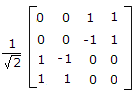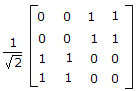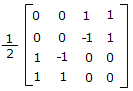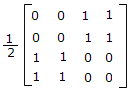# Electronics and Communication Engineering - Microwave Communication

### Exercise :: Microwave Communication - Section 8

11.

In a rectangular waveguide cutoff wavelength for TE10 mode is 8 cm. Then cutoff wavelength for TE20 mode is

 A. 8 cm B. 6 cm C. 4 cm D. 2 cm

Explanation:

No answer description available for this question. Let us discuss.

12.

In a coaxial line

 A. the characteristic impedance is less than that of two wire line B. the wave velocity is less than that of two wire line C. both (a) and (b) D. neither (a) nor (b)

Explanation:

No answer description available for this question. Let us discuss.

13.

If a and b are the width and height of rectangular wave-guide the cut off wavelength λc for TE20 mode is equal to

 A. a B. a + b C. a - b D. a2 + b2

Explanation:

No answer description available for this question. Let us discuss.

14.

A TEM wave is incident normally on a perfect conductor. The E and H fields at boundary will respectively be

 A. minimum and maximum B. minimum and minimum C. maximum and minimum D. maximum and maximum

Explanation:

No answer description available for this question. Let us discuss.

15.

The scattering matrix of magic tee is

 A.B.C.D.